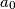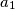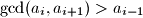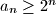### IMO Shortlist 2008 problem N3

Kvaliteta:
Avg: 0,0
Težina:
Avg: 7,0
Let$a_0$,$a_1$,$a_2$,$\ldots$ be a sequence of positive integers such that the greatest common divisor of any two consecutive terms is greater than the preceding term; in symbols,$\gcd (a_i, a_{i + 1}) > a_{i - 1}$. Prove that$a_n\ge 2^n$ for all$n\ge 0$.

Proposed by Morteza Saghafian, Iran
Izvor: Međunarodna matematička olimpijada, shortlist 2008